13 Gravitation

13.3 Gravitational Potential Energy and Total Energy

Learning Objectives

By the end of this section, you will be able to:

• Determine changes in gravitational potential energy over great distances
• Apply conservation of energy to determine escape velocity
• Determine whether astronomical bodies are gravitationally bound

We studied gravitational potential energy in Potential Energy and Conservation of Energy, where the value of g remained constant. We now develop an expression that works over distances such that g is not constant. This is necessary to correctly calculate the energy needed to place satellites in orbit or to send them on missions in space.

Gravitational Potential Energy beyond Earth

We defined work and potential energy in Work and Kinetic Energy and Potential Energy and Conservation of Energy. The usefulness of those definitions is the ease with which we can solve many problems using conservation of energy. Potential energy is particularly useful for forces that change with position, as the gravitational force does over large distances. In Potential Energy and Conservation of Energy, we showed that the change in gravitational potential energy near Earth’s surface is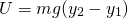. This works very well if g does not change significantly between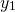and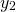. We return to the definition of work and potential energy to derive an expression that is correct over larger distances.

Recall that work (W) is the integral of the dot product between force and distance. Essentially, it is the product of the component of a force along a displacement times that displacement. We define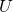as the negative of the work done by the force we associate with the potential energy. For clarity, we derive an expression for moving a mass m from distance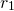from the center of Earth to distance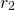. However, the result can easily be generalized to any two objects changing their separation from one value to another.

Consider (Figure), in which we take m from a distancefrom Earth’s center to a distance that isfrom the center. Gravity is a conservative force (its magnitude and direction are functions of location only), so we can take any path we wish, and the result for the calculation of work is the same. We take the path shown, as it greatly simplifies the integration. We first move radially outward from distanceto distance, and then move along the arc of a circle until we reach the final position. During the radial portion,is opposite to the direction we travel along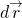, so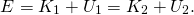Along the arc,is perpendicular to, so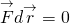. No work is done as we move along the arc. Using the expression for the gravitational force and noting the values for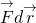along the two segments of our path, we have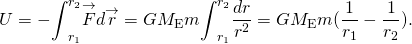Since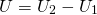, we can adopt a simple expression for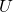: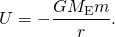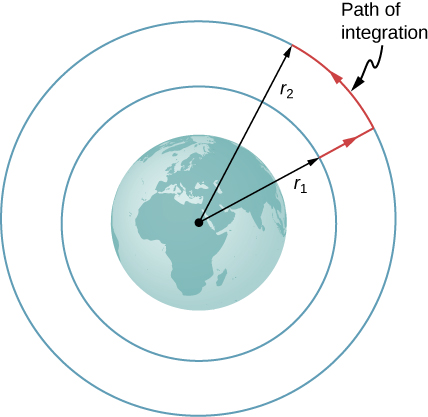Figure 13.11 The work integral, which determines the change in potential energy, can be evaluated along the path shown in red.

Note two important items with this definition. First,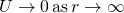. The potential energy is zero when the two masses are infinitely far apart. Only the difference in U is important, so the choice ofis merely one of convenience. (Recall that in earlier gravity problems, you were free to take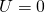at the top or bottom of a building, or anywhere.) Second, note that U becomes increasingly more negative as the masses get closer. That is consistent with what you learned about potential energy in Potential Energy and Conservation of Energy. As the two masses are separated, positive work must be done against the force of gravity, and hence, U increases (becomes less negative). All masses naturally fall together under the influence of gravity, falling from a higher to a lower potential energy.

Example

How much energy is required to lift the 9000-kg Soyuz vehicle from Earth’s surface to the height of the ISS, 400 km above the surface?

Strategy

Use (Figure) to find the change in potential energy of the payload. That amount of work or energy must be supplied to lift the payload.

Solution

Paying attention to the fact that we start at Earth’s surface and end at 400 km above the surface, the change in U is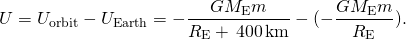We insert the values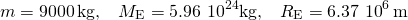and convert 400 km into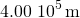. We find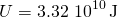. It is positive, indicating an increase in potential energy, as we would expect.

Significance

For perspective, consider that the average US household energy use in 2013 was 909 kWh per month. That is energy of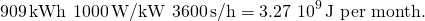So our result is an energy expenditure equivalent to 10 months. But this is just the energy needed to raise the payload 400 km. If we want the Soyuz to be in orbit so it can rendezvous with the ISS and not just fall back to Earth, it needs a lot of kinetic energy. As we see in the next section, that kinetic energy is about five times that of. In addition, far more energy is expended lifting the propulsion system itself. Space travel is not cheap.

Why not use the simpler expression? How significant would the error be? (Recall the previous result, in (Figure), that the value g at 400 km above the Earth is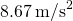.)

The value of g drops by about 10% over this change in height. Sowill give too large a value. If we use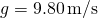, then we get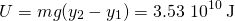which is about 6% greater than that found with the correct method.

Conservation of Energy

In Potential Energy and Conservation of Energy, we described how to apply conservation of energy for systems with conservative forces. We were able to solve many problems, particularly those involving gravity, more simply using conservation of energy. Those principles and problem-solving strategies apply equally well here. The only change is to place the new expression for potential energy into the conservation of energy equation,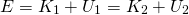.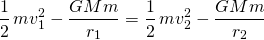Note that we use M, rather than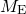, as a reminder that we are not restricted to problems involving Earth. However, we still assume that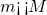. (For problems in which this is not true, we need to include the kinetic energy of both masses and use conservation of momentum to relate the velocities to each other. But the principle remains the same.)

Escape velocity

Escape velocity is often defined to be the minimum initial velocity of an object that is required to escape the surface of a planet (or any large body like a moon) and never return. As usual, we assume no energy lost to an atmosphere, should there be any.

Consider the case where an object is launched from the surface of a planet with an initial velocity directed away from the planet. With the minimum velocity needed to escape, the object would just come to rest infinitely far away, that is, the object gives up the last of its kinetic energy just as it reaches infinity, where the force of gravity becomes zero. Since, this means the total energy is zero. Thus, we find the escape velocity from the surface of an astronomical body of mass M and radius R by setting the total energy equal to zero. At the surface of the body, the object is located at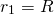and it has escape velocity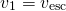. It reaches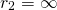with velocity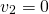. Substituting into (Figure), we have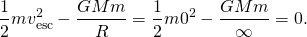Solving for the escape velocity,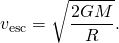Notice that m has canceled out of the equation. The escape velocity is the same for all objects, regardless of mass. Also, we are not restricted to the surface of the planet; R can be any starting point beyond the surface of the planet.

Example

Escape from Earth

What is the escape speed from the surface of Earth? Assume there is no energy loss from air resistance. Compare this to the escape speed from the Sun, starting from Earth’s orbit.

Strategy

We use (Figure), clearly defining the values of R and M. To escape Earth, we need the mass and radius of Earth. For escaping the Sun, we need the mass of the Sun, and the orbital distance between Earth and the Sun.

Solution

Substituting the values for Earth’s mass and radius directly into (Figure), we obtain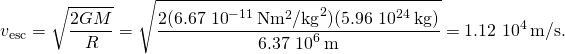That is about 11 km/s or 25,000 mph. To escape the Sun, starting from Earth’s orbit, we use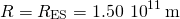and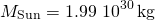. The result is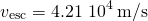Significance

The speed needed to escape the Sun (leave the solar system) is nearly four times the escape speed from Earth’s surface. But there is help in both cases. Earth is rotating, at a speed of nearly 1.7 km/s at the equator, and we can use that velocity to help escape, or to achieve orbit. For this reason, many commercial space companies maintain launch facilities near the equator. To escape the Sun, there is even more help. Earth revolves about the Sun at a speed of approximately 30 km/s. By launching in the direction that Earth is moving, we need only an additional 12 km/s. The use of gravitational assist from other planets, essentially a gravity slingshot technique, allows space probes to reach even greater speeds. In this slingshot technique, the vehicle approaches the planet and is accelerated by the planet’s gravitational attraction. It has its greatest speed at the closest point of approach, although it decelerates in equal measure as it moves away. But relative to the planet, the vehicle’s speed far before the approach, and long after, are the same. If the directions are chosen correctly, that can result in a significant increase (or decrease if needed) in the vehicle’s speed relative to the rest of the solar system.

If we send a probe out of the solar system starting from Earth’s surface, do we only have to escape the Sun?

The probe must overcome both the gravitational pull of Earth and the Sun. In the second calculation of our example, we found the speed necessary to escape the Sun from a distance of Earth’s orbit, not from Earth itself. The proper way to find this value is to start with the energy equation, (Figure), in which you would include a potential energy term for both Earth and the Sun.

Energy and gravitationally bound objects

As stated previously, escape velocity can be defined as the initial velocity of an object that can escape the surface of a moon or planet. More generally, it is the speed at any position such that the total energy is zero. If the total energy is zero or greater, the object escapes. If the total energy is negative, the object cannot escape. Let’s see why that is the case.

As noted earlier, we see that. If the total energy is zero, then as m reaches a value of r that approaches infinity, U becomes zero and so must the kinetic energy. Hence, m comes to rest infinitely far away from M. It has “just escaped” M. If the total energy is positive, then kinetic energy remains at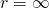and certainly m does not return. When the total energy is zero or greater, then we say that m is not gravitationally bound to M.

On the other hand, if the total energy is negative, then the kinetic energy must reach zero at some finite value of r, where U is negative and equal to the total energy. The object can never exceed this finite distance from M, since to do so would require the kinetic energy to become negative, which is not possible. We say m is gravitationally bound to M.

We have simplified this discussion by assuming that the object was headed directly away from the planet. What is remarkable is that the result applies for any velocity. Energy is a scalar quantity and hence (Figure) is a scalar equation—the direction of the velocity plays no role in conservation of energy. It is possible to have a gravitationally bound system where the masses do not “fall together,” but maintain an orbital motion about each other.

We have one important final observation. Earlier we stated that if the total energy is zero or greater, the object escapes. Strictly speaking, (Figure) and (Figure) apply for point objects. They apply to finite-sized, spherically symmetric objects as well, provided that the value for r in (Figure) is always greater than the sum of the radii of the two objects. If r becomes less than this sum, then the objects collide. (Even for greater values of r, but near the sum of the radii, gravitational tidal forces could create significant effects if both objects are planet sized. We examine tidal effects in Tidal Forces.) Neither positive nor negative total energy precludes finite-sized masses from colliding. For real objects, direction is important.

Example

How Far Can an Object Escape?

Let’s consider the preceding example again, where we calculated the escape speed from Earth and the Sun, starting from Earth’s orbit. We noted that Earth already has an orbital speed of 30 km/s. As we see in the next section, that is the tangential speed needed to stay in circular orbit. If an object had this speed at the distance of Earth’s orbit, but was headed directly away from the Sun, how far would it travel before coming to rest? Ignore the gravitational effects of any other bodies.

Strategy

The object has initial kinetic and potential energies that we can calculate. When its speed reaches zero, it is at its maximum distance from the Sun. We use (Figure), conservation of energy, to find the distance at which kinetic energy is zero.

Solution

The initial position of the object is Earth’s radius of orbit and the intial speed is given as 30 km/s. The final velocity is zero, so we can solve for the distance at that point from the conservation of energy equation. Using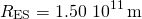and, we have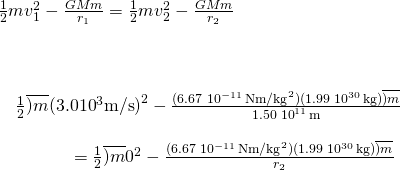where the mass m cancels. Solving forwe get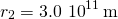. Note that this is twice the initial distance from the Sun and takes us past Mars’s orbit, but not quite to the asteroid belt.

Significance

The object in this case reached a distance exactly twice the initial orbital distance. We will see the reason for this in the next section when we calculate the speed for circular orbits.

Assume you are in a spacecraft in orbit about the Sun at Earth’s orbit, but far away from Earth (so that it can be ignored). How could you redirect your tangential velocity to the radial direction such that you could then pass by Mars’s orbit? What would be required to change just the direction of the velocity?

You change the direction of your velocity with a force that is perpendicular to the velocity at all points. In effect, you must constantly adjust the thrusters, creating a centripetal force until your momentum changes from tangential to radial. A simple momentum vector diagram shows that the net change in momentum is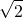times the magnitude of momentum itself. This turns out to be a very inefficient way to reach Mars. We discuss the most efficient way in Kepler’s Laws of Planetary Motion.

Summary

• The acceleration due to gravity changes as we move away from Earth, and the expression for gravitational potential energy must reflect this change.
• The total energy of a system is the sum of kinetic and gravitational potential energy, and this total energy is conserved in orbital motion.
• Objects must have a minimum velocity, the escape velocity, to leave a planet and not return.
• Objects with total energy less than zero are bound; those with zero or greater are unbounded.

Conceptual Questions

It was stated that a satellite with negative total energy is in a bound orbit, whereas one with zero or positive total energy is in an unbounded orbit. Why is this true? What choice for gravitational potential energy was made such that this is true?

It was shown that the energy required to lift a satellite into a low Earth orbit (the change in potential energy) is only a small fraction of the kinetic energy needed to keep it in orbit. Is this true for larger orbits? Is there a trend to the ratio of kinetic energy to change in potential energy as the size of the orbit increases?

As we move to larger orbits, the change in potential energy increases, whereas the orbital velocity decreases. Hence, the ratio is highest near Earth’s surface (technically infinite if we orbit at Earth’s surface with no elevation change), moving to zero as we reach infinitely far away.

Problems

Find the escape speed of a projectile from the surface of Mars.

Find the escape speed of a projectile from the surface of Jupiter.

What is the escape speed of a satellite located at the Moon’s orbit about Earth? Assume the Moon is not nearby.

1440 m/s

(a) Evaluate the gravitational potential energy between two 5.00-kg spherical steel balls separated by a center-to-center distance of 15.0 cm. (b) Assuming that they are both initially at rest relative to each other in deep space, use conservation of energy to find how fast will they be traveling upon impact. Each sphere has a radius of 5.10 cm.

An average-sized asteroid located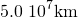from Earth with mass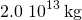is detected headed directly toward Earth with speed of 2.0 km/s. What will its speed be just before it hits our atmosphere? (You may ignore the size of the asteroid.)

(a) What will be the kinetic energy of the asteroid in the previous problem just before it hits Earth? b) Compare this energy to the output of the largest fission bomb, 2100 TJ. What impact would this have on Earth?

(a) What is the change in energy of a 1000-kg payload taken from rest at the surface of Earth and placed at rest on the surface of the Moon? (b) What would be the answer if the payload were taken from the Moon’s surface to Earth? Is this a reasonable calculation of the energy needed to move a payload back and forth?

a.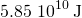; b.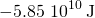; No. It assumes the kinetic energy is recoverable. This would not even be reasonable if we had an elevator between Earth and the Moon.

Glossary

escape velocity
initial velocity an object needs to escape the gravitational pull of another; it is more accurately defined as the velocity of an object with zero total mechanical energy
gravitationally bound
two object are gravitationally bound if their orbits are closed; gravitationally bound systems have a negative total mechanical energy